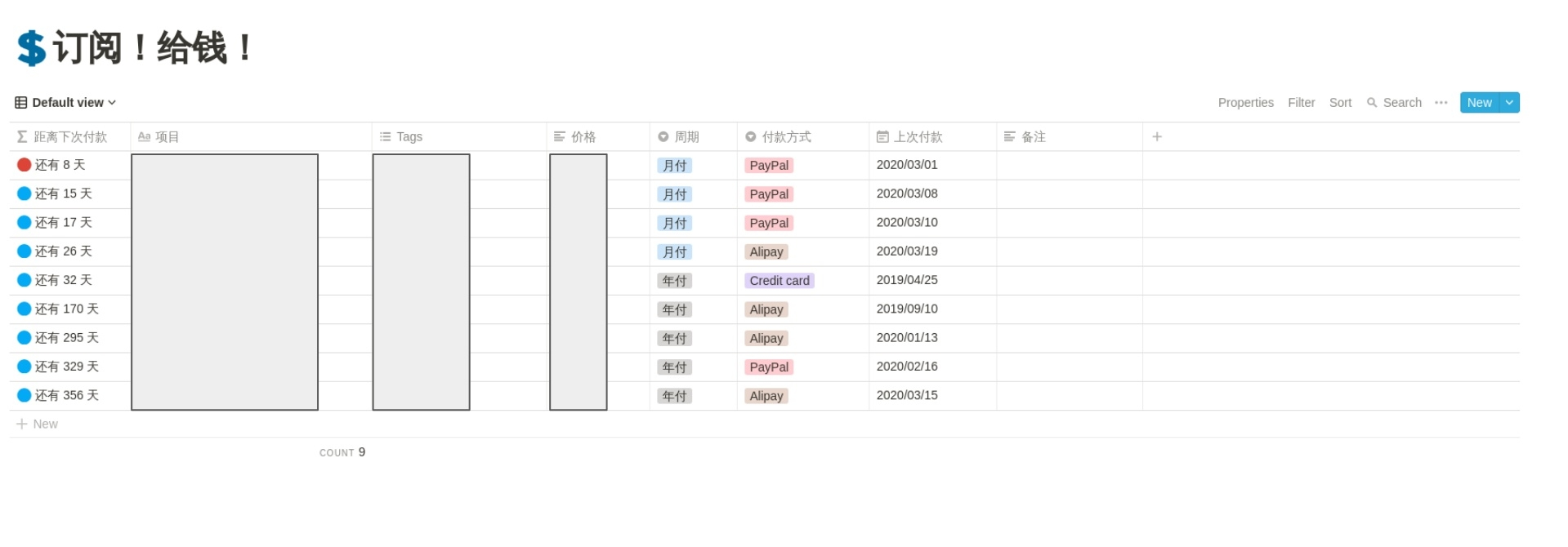2020年的春节是我过得最“憋屈”的一次春节，整整一个月我除了必要的出门以外从大年初一开始我就一直待在了家里，更令我觉得“绝望”的是因为疫情原因我还没法回学校。。。

# 2``````if(prop("周期") == "月付", if(dateBetween(dateAdd(prop("上次付款"), 1, "months"), now(), "days") <= 10, "🔴 还有 " + format(dateBetween(dateAdd(prop("上次付款"), 1, "months"), now(), "days")) + " 天", "🔵 还有 " + format(dateBetween(dateAdd(prop("上次付款"), 1, "months"), now(), "days")) + " 天"), if(dateBetween(dateAdd(prop("上次付款"), 1, "years"), now(), "days") <= 10, "🔴 还有 " + format(dateBetween(dateAdd(prop("上次付款"), 1, "years"), now(), "days")) + " 天", "🔵 还有 " + format(dateBetween(dateAdd(prop("上次付款"), 1, "years"), now(), "days")) + " 天"))
``````